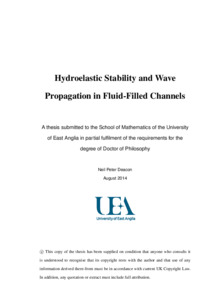# Hydroelastic stability and wave propagation in fluid-filled channels

Deacon, Neil P (2014) Hydroelastic stability and wave propagation in fluid-filled channels. Doctoral thesis, University of East Anglia.Preview
PDF

## Abstract

In this thesis analytical and numerical methods are used to investigate the stability and propagation of waves in various fluid-filled elastic-walled channels. In Chapter 2 a linear temporal stability analysis is performed for various channel wall configurations, the results of which are compared to determine how different physical effects contribute to the stability. In Chapter 3 a linear spatial stability analysis is performed for flow between
linear plates, this is a limiting case of the wall configuration in Chapter 2. Roots of the dispersion relation are found and their stability and direction of propagation determined.

Chapters 4 and 5 focus on the propagation of nonlinear waves on liquid sheets between thin infinite elastic plates. Both linear and nonlinear models are used for the elastic plates. One-dimensional time-dependent equations are derived based on a long wavelength approximation. Symmetric and antisymmetric travelling waves are found
with the linear plate model and symmetric travelling waves are found for the fullynonlinear case. Numerical simulations are employed to study the evolution in time of
initial disturbances and to compare the different models used. Nonlinear effects are found to decrease the travelling wave speed compared with linear models. At sufficiently large amplitude of initial disturbances, higher order temporal oscillations induced by nonlinearity can lead to thickness of the liquid sheet approaching zero. Finally, in Chapter 6, an extension of the model from Chapters 4 and 5 is considered, the elasticities of the two channel walls are allowed to differ. The effect of this difference is determined through a mix of techniques and the limiting case of one wall having no elasticity is discussed.

Item Type: Thesis (Doctoral) Faculty of Science > School of Mathematics Users 2593 not found. 30 Oct 2014 15:32 30 Oct 2014 15:32 https://ueaeprints.uea.ac.uk/id/eprint/50701View Item